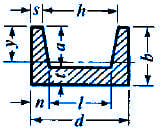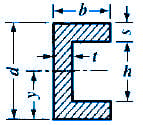Moment of Inertia, Section Modulus, Radii of Gyration Equations
Channel Sections

Moment of Inertia, Section Modulus, Radii of Gyration Equations Channel Sections

 A = Area (in2, mm2) I = Moment of Inertia (in4, mm4) Gr = Radius of Gyration = (in, mm) y = Distance of Axis to Extreme Fiber (in, mm)

 SectionSection Properties Case 28 CalculatorSection Properties Case 29 CalculatorSection Properties Case 30 Calculator Moment of Inertiag = Flange slope (Standard Channels)= 1/6g = Flange slope (Standard Channels)Radius of GyrationAreaySectionSection Properties Case 31 Calculator Moment of InertiaRadius of GyrationAreayRelated:Membership Register | LoginHomeEngineering Book StoreEngineering ForumExcel App. DownloadsOnline Books & ManualsEngineering NewsEngineering VideosEngineering CalculatorsEngineering ToolboxGD&T Training Geometric Dimensioning TolerancingDFM DFA TrainingTraining Online EngineeringAdvertising CenterCopyright Notice